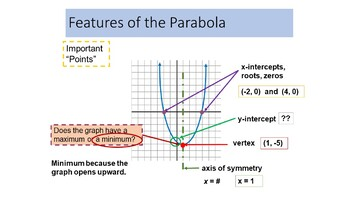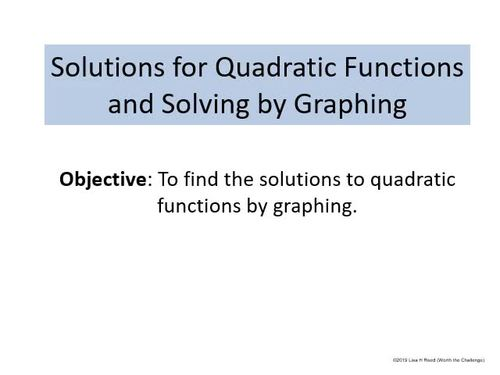# Quadratic Funtions Unit: 9 PPT Lessons with Student Notes - editable versionSubject
Grade Levels
Resource Type
Product Rating
File Type

Compressed Zip File

Be sure that you have an application to open this file type before downloading and/or purchasing.

5 MB|110 pages
Share
Product Description

Algebra 1 Quadratic Functions Unit

This zip file includes all the powerpoints for the lessons and student notes for the Quadratic Functinos unit. All powerpoints and student notes are editable. To purchase "show" powerpoints and print only student notes, please click here.

The accompanying students notes are in powerpoints with animation and answers removed. They may be printed using the print function in powerpoint.

Animation is heavily used in the lessons to help students understand and visualize the concepts. These powerpoints are well suited towards the student that may need more time and practice.

The lessons and student notes included in this zip file are:

Lesson 1 - Exploring Quadratic Graphs

• demonstrates and teaches the key points of a parabola (x-intercepts, y-intercept, vertex, axis of symmetry)
• explains standard form for a quadratic function
• animates how the "a" coefficient changes the direction and shape of the quadratic graph
• provides student practice in describing a quadratic based on the "a" coefficient (width, direction)
• presents a method for graphing quadratics using only the "a" coefficient. Five points are expected to graph the parabola.
• 3 fully animated graphs are presented for students to practice graphing in the form: y = ax^2

Lesson 2 - Exploring Quadratic Functions and Graphs Using "c"

• describes how the "c" coefficient identifies the location of the y-intercept
• describes how the point (0, c) describes the vertex for functions in the form: y = ax^2 + c
• provides student practice in graphing using 5 points and a T chart
• 6 fully animated graphs are presented for students to practice graphing in the form: y = ax^2 + c

Lesson 3 - Finding the Axis of Symmetry, Vertex and Y-Intercept using a,b,c

• describes the characteristics of the "b" coefficient on the shape and location of the parabola
• animates how to find the equation of the line for the axis of symmetry using b/2a
• animates how to calculate the vertex using the axis of symmetry
• reviews finding the y-intercept
• provides student practice for finding these values for various quadratic functions

Lesson 4 - Graphing y = ax^2 + bx + c

• reviews calculating axis of symmetry, vertex and finding y-intercept
• provides a method for graphing full quadratic functions using an extended T-chart, graphing the axis of symmetry and finding 5 major points at minimum: vertex, y-intercept and its reflexive match, a third calculated point and its reflexive match.
• 6 fully animated graphs are presented for students to practice

Lesson 5 - Solutions for Quadratic Functions and Solving by Graphing

• describes the difference between a quadratic function and equation and presents some applications examples in real life
• teaches to the concept of a "solution" (x-intercepts, the zeros or roots) of a quadratic function or equation
• provides more graphing practice with the aim at finding the solutions using graphs

Lesson 6 - Solving Using the Square Root Method

• reviews how to find both positive and negative square roots
• reviews factoring special case, difference of the squares
• teaches solving quadratic equations in the form of 0 = ax^2 + c using the Square Root method
• provides practice for this new method

Lesson 7 - REVIEW: Solving by Factoring

• review solving using just factoring of all types. Students should have completed the Factoring Unit prior to this lesson. Students must recognize if the equation is already in factored form or if it needs to be factored before finding the x-intercepts.

Lesson 8 - Solving Using the Quadratic Formula

• introduces quadratic equations/functions that cannot be solve with methods learned so far and must be solved using the Quadratic Formula
• animates using the quadratic formula in steps - first by finding the discriminant and then using the complete formula
• 12 fully animated problems are presented for students to practice

Lesson 9 - Solving Applications of Quadratic Functions

• animates solving various applications of quadratic functions
• Examples include: consecutive number problems, geometric problems, Pythagorean Theorem problems and Vertical Motion problems
• The problems use a mixture of all solution methods (factoring, square root and quadratic formula)

This package includes over 120 slides and over 110 problems.

These are more quadratic word problems using various methods:

Note: The thumbnails come from different lessons in this unit. The preview is an example of the powerpoints animation and quality for Lesson 5. The movie shows a preview of Lesson 5.

Licensing: This purchase includes a license for one teacher only for personal use in their classroom. Licenses are non-transferable, meaning they can not be passed from one teacher to another. No part of this resource is to be shared with colleagues or used by an entire grade level, school, or district without purchasing the proper number of licenses.

Copywrite terms: This resource may not be uploaded to the internet in any form, including classroom/personal websites or network drives, unless the site is password protected and can only be accessed by students.

Total Pages
110 pages
Answer Key
N/A
Teaching Duration
2 Weeks
Report this ResourceSign Up